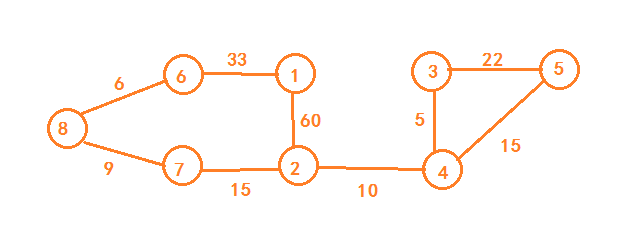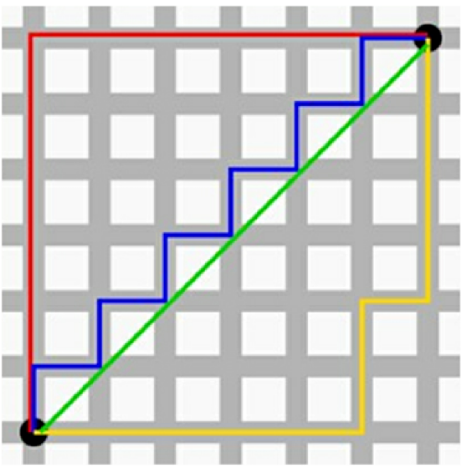# A-star 算法原理分析

## A* 算法

A* 算法实际上是对 Dijkstra 算法的优化和改造后得到的。Dijkstra 算法详见：

Dijkstra 算法的核心思想是每次都获取离起始点最近的节点，再向外扩展。f(i) = g(i) + h(i)


## A* 算法的代码实现

public class Graph {

private class Edge {// 表示边
public int sid;// 边的起始节点
public int tid;// 边的结束节点
public int w;// 边的权重

public Edge(int s, int t, int w) {
this.sid = s;
this.tid = t;
this.w = w;
}
}

private class Vertex {
public int id; // 顶点编号 ID
public int dist; // 从起始顶点，到这个顶点的距离，也就是 g(i)
public int f; // 新增：f(i)=g(i)+h(i)
public int x, y; // 新增：顶点在地图中的坐标（x, y）
public Vertex(int id, int x, int y) {
this.id = id;
this.x = x;
this.y = y;
this.f = Integer.MAX_VALUE;
this.dist = Integer.MAX_VALUE;
}
}

private Vertex[] vertexes;//记录所有顶点的坐标
private int v;// 顶点数

public Graph(int v) {
this.v = v;
Vertex[] vertexes = new Vertex[this.v];
for(int i; i<v; i++) {
}
}

public void addEdge(int s, int t, int w) {
}

//添加顶点坐标
public void addVetex(int id, int x, int y) {
vertexes[id] = new Vertex(id, x, y)
}
}



1. 图类中增加成员变量Vertex[] vertexes记录所有节点的坐标
2. 构造函数中进行初始化Vertex[] vertexes
3. 新增添加节点坐标的方法addVetex(int id, int x, int y)

1. 优先队列的构建方式不同，A* 算法是使用代码中public int f;这个值来构建的，而 Dijkstra 算法是用 dist 值构建的
2. A* 算法除了需要更新 dist 值，还需要更新 f 值。
3. A* 算法循环结束的条件是只要遍历到终点即退出循环，因此 A* 算法最终并不一定能得到最短路径。
public void AStar(Graph g, int s, int t) { // 从顶点 s 到顶点 t 的路径
int[] predecessor = new int[this.v]; // 用来还原路径

// 按照 vertex 的 f 值构建的小顶堆，而不是按照 dist
PriorityQueue queue = new PriorityQueue(this.v);

boolean[] inqueue = new boolean[this.v]; // 标记是否已经走过

// 起始节点初始化
vertexes[s].dist = 0;
vertexes[s].f = 0;
inqueue[s] = true;

while (!queue.isEmpty()) {
Vertex minVertex = queue.poll(); // 取堆顶元素并删除
for (int i=0; i<g.adj[minVertex.id].size(); i++) {
Edge e = g.adj[minVertex.id].get(i); // 取出一条 minVetex 相连的边
Vertex nextVertex = vertexes[e.tid]; // 下一个节点
if (minVertex.dist + e.w < nextVertex.dist) { // 更新 next 的 dist,f

nextVertex.dist = minVertex.dist + e.w;// 更新 dist
nextVertex.f = nextVertex.dist+hManhattan(nextVertex, vertexes[t]);// 更新 f 值

// 记录路径
predecessor[nextVertex.id] = minVertex.id;

//更新队列中的节点信息
if (inqueue[nextVertex.id] == true) {
queue.update(nextVertex);
} else {
inqueue[nextVertex.id] = true;
}
}
if (nextVertex.id == t) { // 只要到达终点就退出循环
queue.clear(); // 清空队列，否则无法退出 while 循环
break;
}
}
}
// 输出路径
System.out.print(s);
print(s, t, predecessor);
}

/**
* 计算曼哈顿距离
*
*/
int hManhattan(Vertex v1, Vertex v2) {
return Math.abs(v1.x - v2.x) + Math.abs(v1.y - v2.y);
}

private void print(int s, int t, int[] pre) {
if(s == t) return;
print(s, pre[t], pre);
System.out.print("->"+t);
}



## 拓展

A* 算法属于**启发式搜索算法（Heuristically Search Algorithm）**的一种，其他属于启发式搜索的算法还有：IDA* 算法、蚁群算法、遗传算法、模拟退火算法等等。

08-238万+06-11164
05-064602
04-094238
11-099465
09-015382
04-114482
02-194万+
11-10
10-152562